# MCAT General Chemistry Practice Test 1: Atomic Structure

### Test Information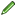15 questions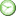minutes

Take more free MCAT general chemistry practice tests available from maintests.com.

1. Which of the following is the correct electron configuration for Zn2+?

• A. 1s22s22p63s23p64s03d10
• B. 1s22s22p63s23p64s23d8
• C. 1s22s22p63s23p64s23d10
• D. 1s22s22p63s23p64s03d8

2. Which of the following quantum number sets is possible?

• A. n = 2; l = 2; ml = 1;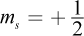• B. n = 2; l = 1; ml = -1;• C. n = 2; l = 0; ml = -1;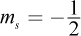• D. n = 2; l = 0; ml = 1;3. What is the maximum number of electrons allowed in a single atomic energy level in terms of the principal quantum number n?

• A. 2n
• B. 2n + 2
• C. 2n2
• D. 2n2 + 2

4. Which of the following equations describes the maximum number of electrons that can fill a subshell?

• A. 2l + 2
• B. 4l + 2
• C. 2l2
• D. 2l2 + 2

5. Which of the following atoms only has paired electrons in its ground state?

• A. Sodium
• B. Iron
• C. Cobalt
• D. Helium

6. An electron returns from an excited state to its ground state, emitting a photon at λ = 500 nm. What would be the magnitude of the energy change if one mole of these photons were emitted? (Note: h = 6.626 × 10-34 J·s)

• A. 3.98 × 10-21 J
• B. 3.98 × 10-19 J
• C. 2.39 × 103 J
• D. 2.39 × 105 J

7. Suppose an electron falls from n = 4 to its ground state, n = 1. Which of the following effects is most likely?

• A. A photon is absorbed.
• B. A photon is emitted.
• C. The electron moves into a p-orbital.
• D. The electron moves into a d-orbital.

8. Which of the following isotopes of carbon is LEAST likely to be found in nature?

• A. 6C
• B. 12C
• C. 13C
• D. 14C

9. Which of the following best explains the inability to measure position and momentum exactly and simultaneously according to the Heisenberg uncertainty principle?

• A. Imprecision in the definition of the meter and kilogram
• B. Limits on accuracy of existing scientific instruments
• C. Error in one variable is increased by attempts to measure the other
• D. Discrepancies between the masses of nuclei and of their component particles

10. Which of the following electronic transitions would result in the greatest gain in energy for a single hydrogen electron?

• A. An electron moves from n = 6 to n = 2.
• B. An electron moves from n = 2 to n = 6.
• C. An electron moves from n = 3 to n = 4.
• D. An electron moves from n = 4 to n = 3.

11. Suppose that an atom fills its orbitals as shown: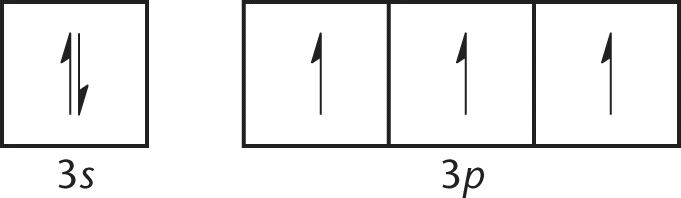Such an electron configuration most clearly illustrates which of the following laws of atomic physics?

• A. Hund's rule
• B. Heisenberg uncertainty principle
• C. Bohr model
• D. Rutherford model

12. How many total electrons are in a 133Cs cation?

• A. 54
• B. 55
• C. 78
• D. 133

13. The atomic weight of hydrogen is 1.008 amu. What is the percent composition of hydrogen by isotope, assuming that hydrogen's only isotopes are 1H and 2D?

• A. 92% H, 8% D
• B. 99.2% H, 0.8% D
• C. 99.92% H, 0.08% D
• D. 99.992% H, 0.008% D

14. Consider the two sets of quantum numbers shown in the table, which describe two different electrons in the same atom.

 n l ml ms 2 1 1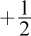3 1 -1Which of the following terms best describes these two electrons?

• A. Parallel
• B. Opposite
• C. Antiparallel
• D. Paired

15. Which of the following species is represented by the electron configuration 1s22s22p63s23p64s13d5?

I. Cr

II. Mn+

III. Fe2+

• A. I only
• B. I and II only
• C. II and III only
• D. I, II, and III

﻿